### How to Solve: CFOP Method

CFOP is an advanced method for solving the Rubik's Cube. The main method for solving the Rubik's Cube learnt by beginners is aptly named the "Beginners Method". In this article we will be talking about the different sages of the CFOP method and how to solve your Rubik's Cube using the CFOP method.

CFOP stands for CROSS > F2L > OLL > PLL - We will discuss this more later on.

### F2L ALGORITHMS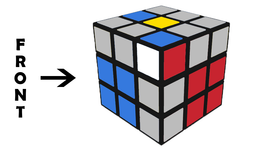### OLL ALGORITHMS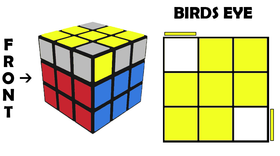### PLL ALGORITHMS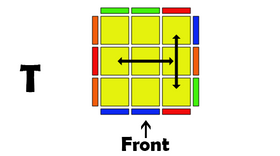### Step 1 - Cross

In step 1 we concentrate on building the cross. Its super easy and intuitive and if you can solve your cube with the beginners method then you should be able to solve it now without a guide.

### Step 2 - F2L

In step 2 we solve the first 2 layers (F2L). We do this by inserting the first layer corner and the 2nd layer edge piece in 1 algorithm.

### Step 3 - OLL

In step 3 we orientate all of the last layer. Basically, we bring all of the yellow side to the top layer. There are 57 algorithms to learn FULL OLL.

### Step 4 - PLL

In step 4 we just have to shuffle all of the top face around so that we solve the rest of the puzzle. This is called permuting the last layer. There are only 21 algorithms to learn FULL PLL.

### Corner on Top / Edge on Top (matching)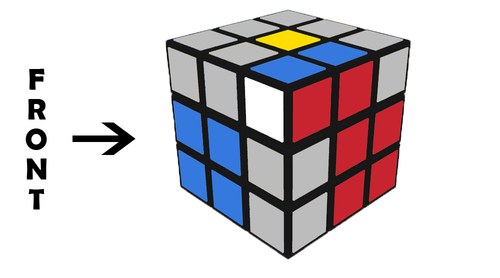U (R U' R)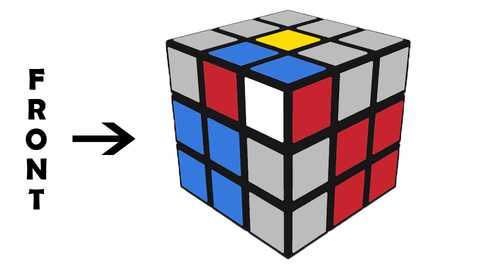y' R' U R U' d' (R U R')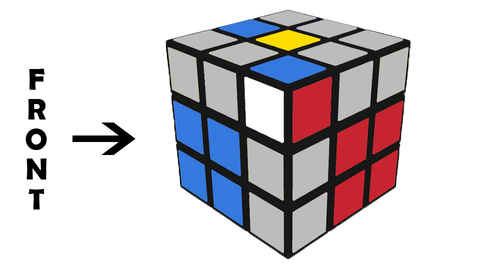U' R U2' R' U2 (R U' R')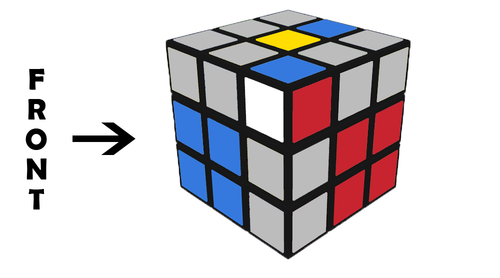(U' R U R') U2 (R U' R')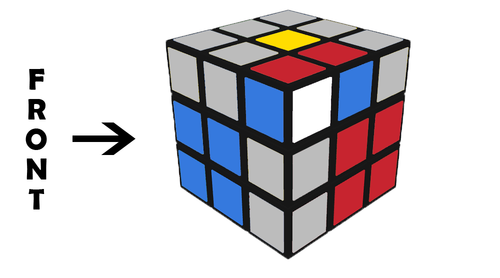(R U' R' U) d (R' U' R)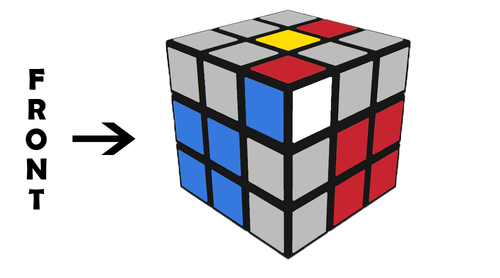d (R' U2 R) U2' (R' U R)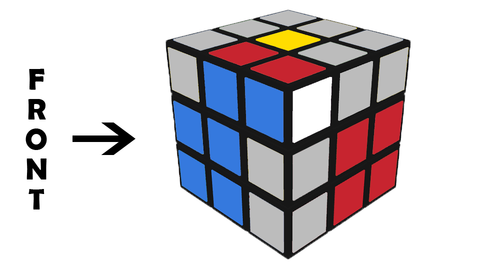y' U' (R' U R)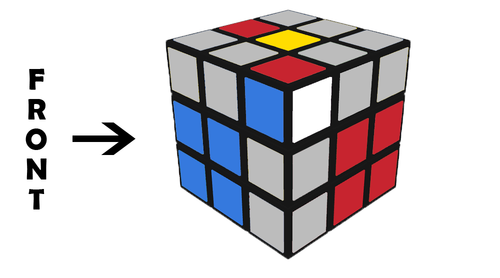d (R' U' R) U2' (R' U R)

### Corner on Top / Edge on Top (opposite)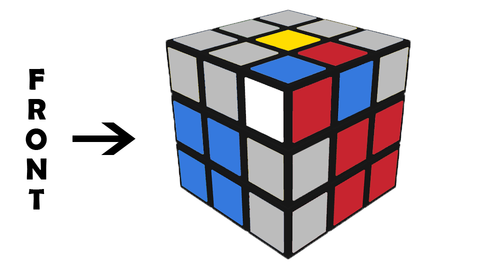U' (R U2' R') d (R' U' R)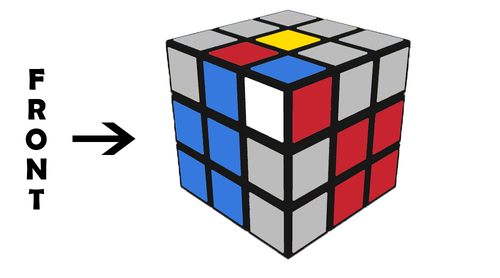d R' U R U' (R' U' R)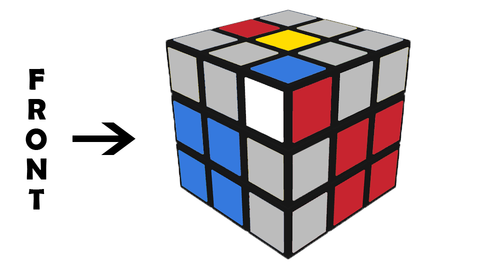y (L' U' L)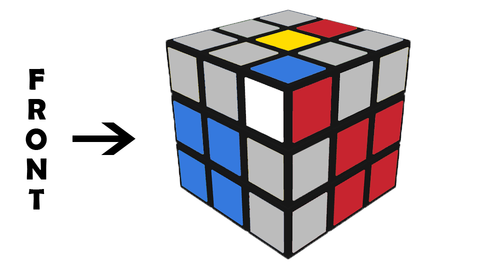(U' R U' R' U) y L' U' L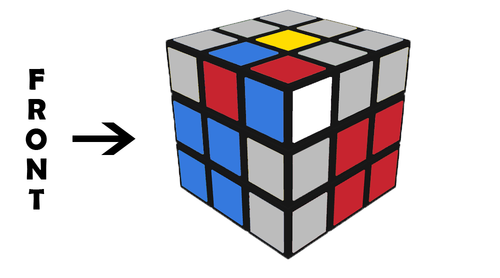R' U2 R2 U R2' U R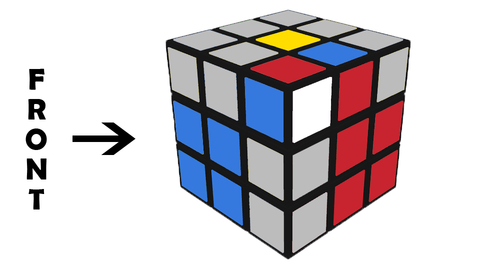U' (R U' R' U) (R U R')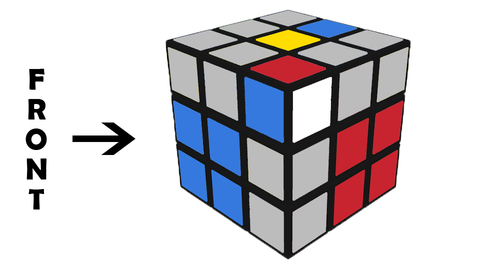(R U R')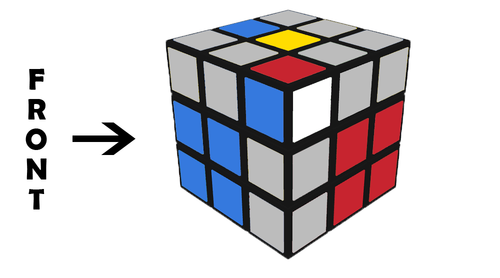U' R U R' U (R U R')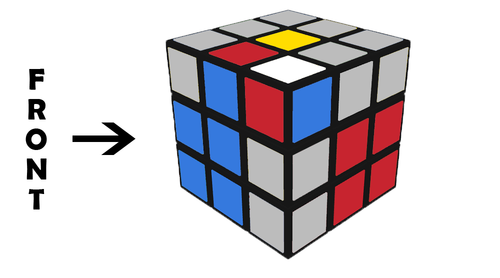U2 (R U R' U)(R U' R')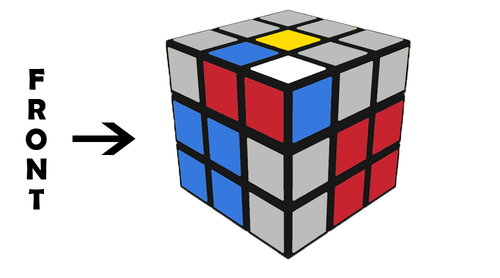U (R' F R F')(R U R') U2 (R U' R')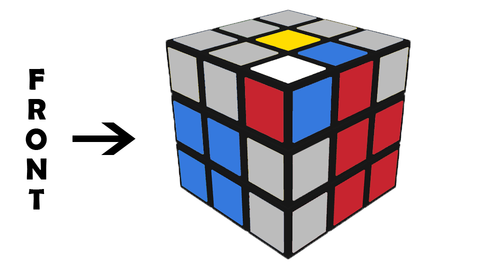(R U2 R') U' (R U R')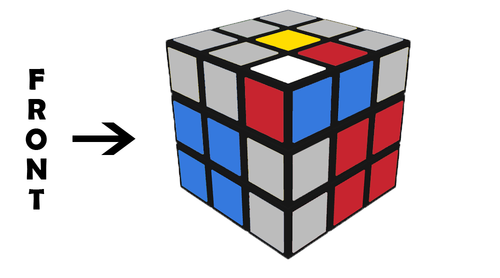(R U' R' U) (R U' R')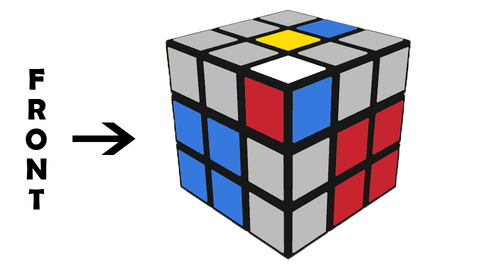U R U2 R' U (R U' R')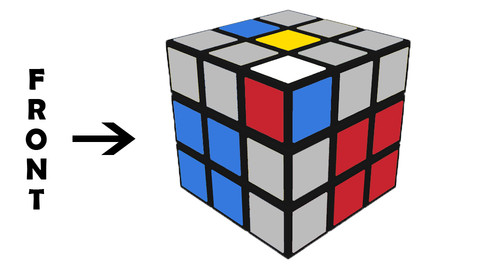U' (F R' F' R) y' (R' U' R) U2 (R' U' R)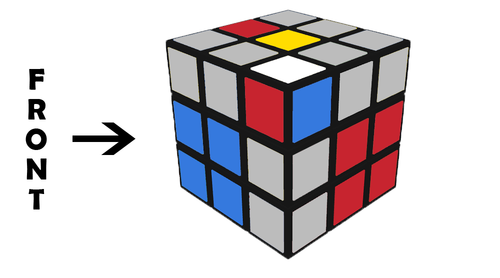y' U' (R' U2 R) U' (R' U R)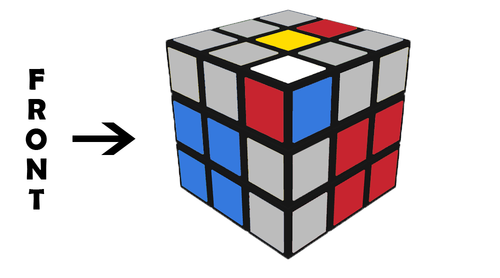y' U2 R' U' R U' (R' U R)

### Corner on Top / Edge on Bottom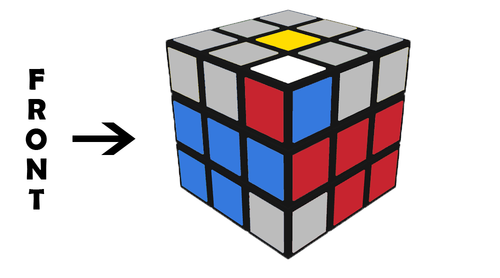(R U R' U')(R U R' U')(R U R')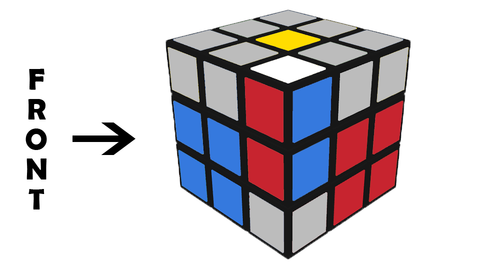U' R' F R F' R U' R'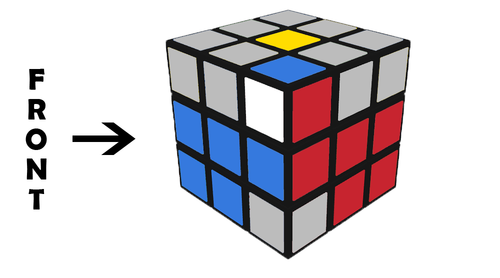U' R U' R' U2 (R U' R')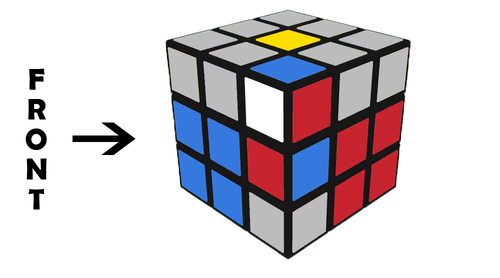(U' R U R') d (R' U' R)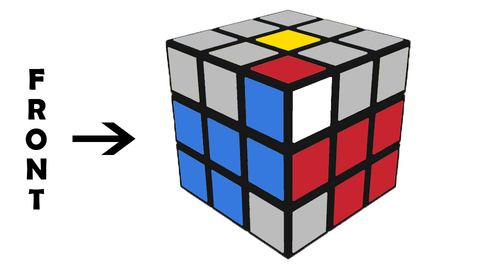U (R U R') U2 (R U R')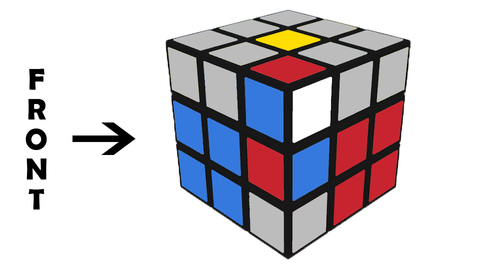d R' U' R d' (R U R')

### Corner on Bottom / Edge on Top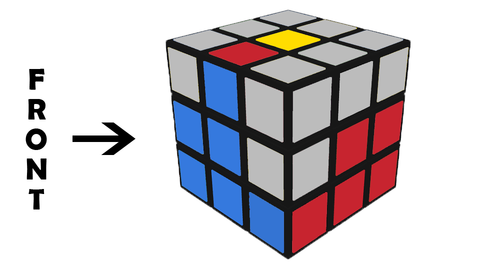U (R U' R') (F R' F' R)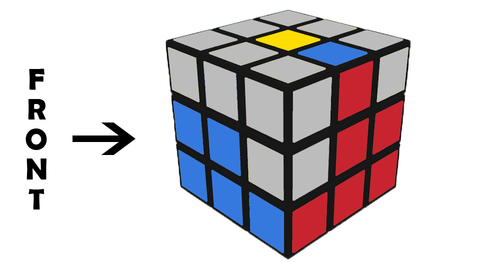y (U' L' U L) y' (U R U' R')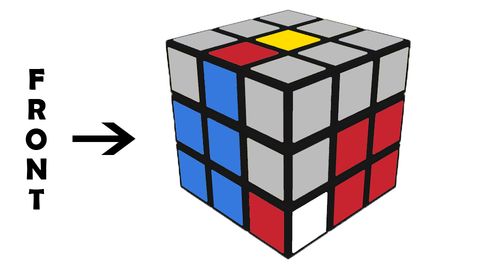(R U R' U') F R' F' R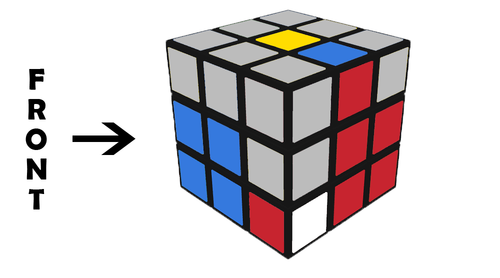R U R' U' (R U R')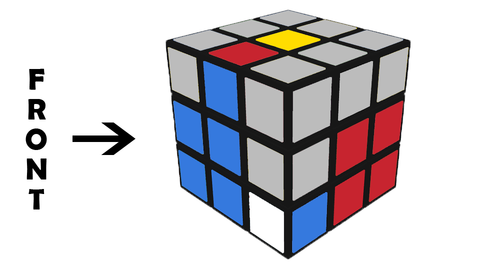y' (R' U' R U)(R' U' R)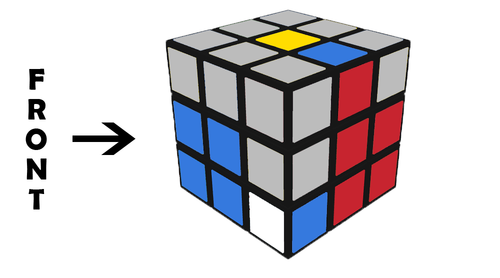(R U' R' U) (R U R')

### Corner on Bottom / Edge on Bottom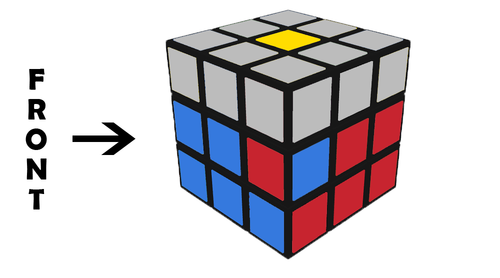(R U' R') d (R' U2 R) U2' (R' U R)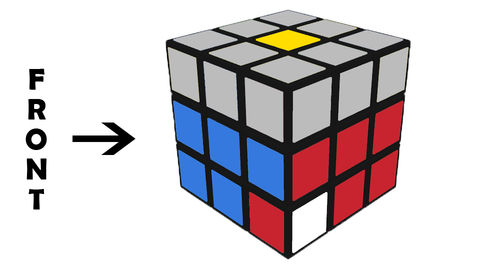(R U R') U2 (R U' R' U)(R U R')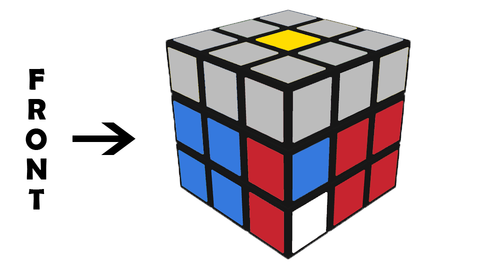(R U' R' U) d (R' U' R U') (R' U R)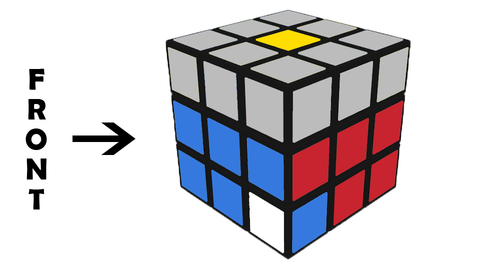R U' R' U' R U R' U2 (R U' R')(R U' R') d (R' U' R U')(R' U' R)

Note: If you think you know a better algorithm please do reach out and let us know.

Section